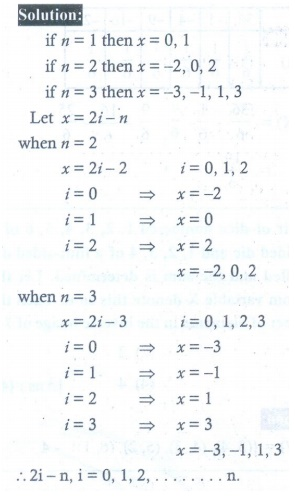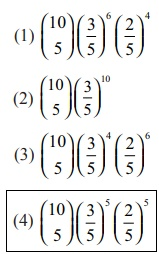Home | | Maths 12th Std | Exercise 11.6: Choose the Correct answer

# Exercise 11.6: Choose the Correct answer

Choose the Correct or the most suitable answer from the given four alternatives - Maths Book back answers and solution for Exercise questions - Problem Questions with Answer, Solution - Maths (Mathematics) : Probability Distributions

EXERCISE 11.6

Choose the Correct or the most suitable answer from the given four alternatives :

1. Let x be random variable with probability density functionWhich of the following statement is correct?

(1) both mean and variance exist

(2) mean exists but variance does not exist

(3) both mean and variance do not exist

(4) variance exists but Mean does not exist.2. A rod of length 2l is broken into two pieces at random. The probability density function of the shorter of the two pieces isThe mean and variance of the shorter of the two pieces are respectively3. Consider a game where the player tosses a six-sided fair die. If the face that comes up is 6, the player wins  36, otherwise he loses  k 2 , where k is the face that comes up k = {1, 2, 3, 4, 5}. The expected amount to win at this game in  is

(1) 19/6

(2) – 19/6

(3) 3/2

(4) – 3/24. A pair of dice numbered 1, 2, 3, 4, 5, 6 of a six-sided die and 1, 2, 3, 4 of a four-sided die is rolled and the sum is determined. Let the random variable X denote this sum. Then the number of elements in the inverse image of 7 is

(1) 1

(2) 2

(3) 3

(4) 45. A random variable X has binomial distribution with n = 25 and p = 0.8 then standard deviation of X is

(1) 6

(2) 4

(3) 3

(4) 26. Let X represent the difference between the number of heads and the number of tails obtained when a coin is tossed n times. Then the possible values of X are

(1) i+2n, i = 0,1,2...n

(2) 2ini = 0,1,2...n

(3) nii = 0,1,2...n

(4) 2i+2ni = 0,1,2...n7. If the function (x) = 1/12 for a < x < b , represents a probability density function of a continuous random variable X, then which of the following cannot be the value of a and b?

(1) 0 and 12

(2) 5 and 17

(3) 7 and 19

(4) 16 and 248. Four buses carrying 160 students from the same school arrive at a football stadium. The buses carry, respectively, 42, 36, 34, and 48 students. One of the students is randomly selected. Let denote the number of students that were on the bus carrying the randomly selected student. One of the 4 bus drivers is also randomly selected. Let Y denote the number of students on that bus.

Then E(X) and E(Y) respectively are

(1) 50, 40

(2) 40, 50

(3) 40.75, 40

(4) 41, 419. Two coins are to be flipped. The first coin will land on heads with probability 0.6, the second with Probability 0.5. Assume that the results of the flips are independent, and let X equal the total number of heads that result. The value of E(X) is

(1) 0.11

(2) 1.1

(3)11

(4)110. On a multiple-choice exam with 3 possible destructives for each of the 5 questions, the probability that a student will get 4 or more correct answers just by guessing is

(1) 11/243

(2) 3/8

(3) 1/243

(4) 5/24311. If P(X = 0) = 1 − P(X = 1). If E(X) = 3Var(X), then P(X = 0) is

(1) 2/3

(2) 2/5

(3) 1/5

(4) 1/312. If X is a binomial random variable with expected value 6 and variance 2.4, then P(X = 5) is13. The random variable x has the probability density functionand E ( x ) = 7/12, then a and b are respectively

(1) 1 and 1/2

(2) 1/2 and 1

(3) 2 and 1

(4) 1 and 214. Suppose that x takes on one of the values 0, 1, and 2. If for some constant k,

P (= i ) = k P ( X = i −1) for i = 1, 2 and P ( x = 0 ) = 1/7 , then the value of is

(1) 1

(2) 2

(3) 3

(4) 415. Which of the following is a discrete random variable?

I. The number of cars crossing a particular signal in a day.

II. The number of customers in a queue to buy train tickets at a moment.

III. The time taken to complete a telephone call.

(1) I and II

(2) II only

(3) III only

(4) II and III

16. Ifis a probability density function of a random variable, then the value of a is

(1) 1

(2) 2

(3) 3

(4) 417. The probability mass function of a random variable is defined as:Then E(X) is equal to:

(1) 1/15

(2) 1/10

(3) 1/3

(4) 2/318. Let X have a Bernoulli distribution with mean 0.4, then the variance of (2X–3) is

(1) 0.24

(2) 0.48

(3) 0.6

(4) 0.9619. If in 6 trials, X is a binomial variable which follows the relation 9P(X=4) = P(X=2), then the probability of success is

(1) 0.125

(2) 0.25

(3) 0.375

(4) 0.7520. A computer salesperson knows from his past experience that he sells computers to one in every twenty customers who enter the showroom. What is the probability that he will sell a computer to exactly two of the next three customers?

(1) 57/203

(2) 57/202

(3) 193/203

(4) 57/20Tags : Probability Distributions | Mathematics , 12th Maths : UNIT 11 : Probability Distributions
Study Material, Lecturing Notes, Assignment, Reference, Wiki description explanation, brief detail
12th Maths : UNIT 11 : Probability Distributions : Exercise 11.6: Choose the Correct answer | Probability Distributions | Mathematics